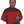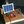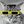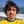0%
0%

# CapSens 3d

An Arduino Powered 3d Capacitive Sensor, using only Aluminum Foil Paper and Resistors

Similar projects worth following
466 views
The Project is a simple 3d Capacitive Sensor which can detect the position of the hand in a 3d Axis system and output the Results in 3d Axis System on a Linux PC (sorry Windows fans).
Stay tuned for the Design Choices and limitations DesBlog, as well as the manual

Video Demo:

The Projects consists of the Software for both the Arduino and the Host PC

Arduino Software:

The Code can be found on the following github page: https://github.com/vpetrog/CapSens_3d

Part of the project's code is a fork of:

CapacitiveSensor library, Copyright (c) 2009 Paul Bagder https://github.com/PaulStoffregen/CapacitiveSensor.

However, the majority of the Library is rewritten and specifically optimized for the needs of the Project.

Host- PC Application

The Application is a python Script utilizing the power of matplotlib library

Dependencies:

• Linux Os (can be easily forked to Windows though)
• Python 3
• Python Modules:
• serial
• numpy
• matplotlip

The script unfortunately lacks comment's, which will be added in the near future

### Video_Demo_1.mp4

MPEG-4 Video - 19.63 MB - 07/04/2017 at 18:18

• 3 × Resistor 4.7 Mohm
• 1 × Arduino Uno Or any other compatible avr microcontroller

• ### DesBlog #1 : Theory of Operation

Evangelos Petrongonas07/04/2017 at 21:53 0 comments

Welcome to the first out of two (or three) DesBlogs, where we will discuss the theory and the construction of the Sensor.

In this first one lets discus about the theory that makes the idea doable.

The theory behind the sensor is pretty simple and straightforward, as it is based on the Capacitor Charging phenomenon.

Firstly let’s remember what affects the Capacitance of a parallel plate system. According to the well-known relation C= (A.er) /d , where A is the area of the plates, er is the dielectric permitivity of the material between the plates and d, is the distance between the plates.

Let’s Consider now that both A and er, are constant. As a result the Capacitance is inversely proportional to the distance. That is very important deduction, as now we know that by measuring the capacitance, we can find the distance between the plates.

Now consider that one plate is a plane of a three dimensional axis, and the other plate is a hand (or any other grounded object). By having three of these plates, placed in a way that that are pair-wise perpendicular, we create a Cartesian coordinate system, where the object can be represented in.

Enough with the Math, at least for now, let’s move to the next big Design Puzzle, which is, how can we measure the Capacitance?

The answer is we don’t…. kind off….

Before we clarify the above statement, let us take about microcontrollers and more precisely, the way they can interact with environment. A uC, can “sense” only three things. Time, Analog Current (using the ADC’s) and Digital Levels 0,1.

As you can see Capacitance is not one of them

BUT,

after some more math we come up to the famous time constant. Time constant (t) is the time that it takes the capacitor to charge up to the 63 % of the supply voltage, when charged through a resistor. More over τ = RC, so τ ~ 1/d. Congratulations ! the distance is now linked to a value that the uC can measure, but we still have to figure out how to find the time constant. One may think to measure the voltage of the capacitor directly using the ADC’s, but there is a more efficient way of doing it by using the RTFM method (if you don’t know what RTFM means, I strongly suggest you to google it. In order to give you a hint R stands for Read…). The Digital Input Pins of the uC are buffered and a Comparator is used in order to determine whether the Sense Voltage level is 0 or 1. If the Anlog (let’s assume DC) input voltage is greater than the threshold voltage the it reads 1, or 0 otherwise. The threshold voltage for the mega328p (Arduino UNO), is 0.6 time the Source voltage, which is very close to the 63 % of the time consant.

Voila, the time constant is equal to the time it take for the input to go HIGH, while this is accomplished simply by connecting directly every plate to an Arduino Pin.

One more step is required and we are ready. In order to display distance we convert the time measured to a normalized distance approximation based on some more math and the Calibration Curves, which luckily you don’t have to make, since we have done them for you :)

In conclusion, everything that is mentioned below can be summed up in the following picture.

Stay tuned for the next DesBlog where we will discuss the Design Choices and limitations.

Until Then,

keep hacking,

Evangelos.

Share

## Similar Projects

Project Owner Contributor

### BPW34 solar powered led watchbobricius

Project Owner Contributor

### Distance Measurement Using Arduino，Utsource viewElectroniclovers123

Project Owner Contributor

### Guri: Ignition Controller for Air Cooled Enginesdanjovic

Project Owner Contributor

### Retrofitting of an old tester from 1955Giulio Pons

# Does this project spark your interest?

Become a member to follow this project and never miss any updates